# Flipped Classroom Lines and Angles

##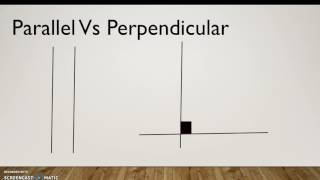By Megan Priole

Draw points, lines, line segments, rays, angles (right, acute, obtuse), and perpendicular and parallel lines.# Math Antics - Points, Lines, & Planes

##By mathanatics

Draw points, lines, line segments, rays, angles (right, acute, obtuse), and perpendicular and parallel lines. Identify these in two-dimensional figures.# 8.5 Three Theorems Involving Proportions (Lesson)

##By AutenMath

A lesson on the Angle Bisector and Side Splitter theorems and a theorem relating segments cut by two transversals and three or more parallel lines.# McGraw-Hill Math Tutoring | MathHelp.com

##By MathHelp.com

This lesson covers the midsegment of triangle. Students learn the following theorems related to parallel lines. If 3 parallel lines form congruent segments along one transversal, then they form congruent segments along every transversal. If a line intersects the midpoint of one side a triangle and is parallel to another side of the triangle, then it intersects the midpoint of the third side of the triangle. If a segment joins the midpoints of two sides of a triangle, then the segment is (a) parallel to the third side of the triangle, and (b) half the length of the third side. Students are then asked to solve problems related to these theorems using Algebra.# Proof: Vertical angles are equal | Angles and intersecting lines | Geometry | Khan Academy

##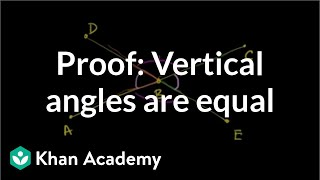By Khan Academy# Complementary and supplementary angles | Angles and intersecting lines | Geometry | Khan Academy

##By Khan Academy# Triangle angle example 1 | Angles and intersecting lines | Geometry | Khan Academy

##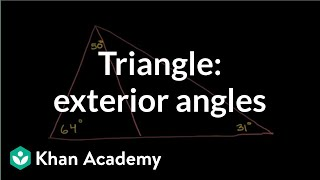By Khan Academy

Figuring out angles in a triangle. A little about exterior angles being the sum of the remote interior angles# Measuring angles in degrees | Angles and intersecting lines | Geometry | Khan Academy

##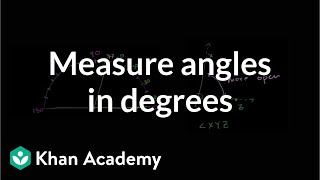By KhanAcademy

exact way to measure an angle using a protractor# Angles (part 3) | Angles and intersecting lines | Geometry | Khan Academy

##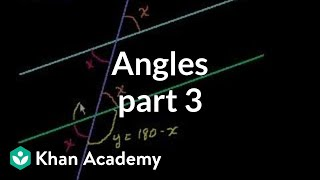By Khan Academy

This video uses an electronic black board with different colored pens. Angles formed with two parallel lines (defines parallel lines) and a transversal line (a line that crosses the two parallel lines). The video then goes into labeling the various angles, corresponding angles, opposite angles, alternate interior angles, alternate exterior angles, and supplementary angles. The last thing the video explains is that all the angles in a triangle add up to 180 degrees. It shows how to find the measurements of each of these angles. Good video for review of concepts and angle vocabulary. Good for middle school students.# Triangle angle example 1 | Angles and intersecting lines | Geometry | Khan Academy

##By Khan Academy

Figuring out angles in a triangle. A little about exterior angles being the sum of the remote interior angles# Step-By-Step Instructions for Geometric Constructions

##By Ezlie

This video gives us each step of the essential geometric constructions. The first part of this video goes through some important geometric vocabulary (parallel lines, midpoint, etc), so you may want to skip ahead to 1:30 to get to the constructions. Constructions in this video: Copying a line segment Construcing the perpendicular bisector of a line segment Constructing a perpendicular to a line through a point not on the line. Constructing a perpendicular to a line through a point on the line. Bisecting an angle Copying an angle# Step-By-Step Instructions for Geometric Constructions

##By Ezlie

This video gives us each step of the essential geometric constructions. The first part of this video goes through some important geometric vocabulary (parallel lines midpoint etc) so you may want to skip ahead to 1:30 to get to the constructions. Constructions in this video: Copying a line segment Construcing the perpendicular bisector of a line segment Constructing a perpendicular to a line through a point not on the line. Constructing a perpendicular to a line through a point on the line. Bisecting an angle Copying an angle# Find measure of vertical angles | Angles and intersecting lines | Geometry | Khan Academy

##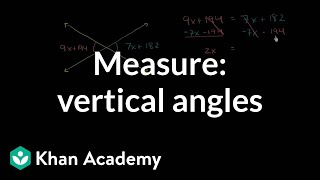By KhanAcademy

Now that we've learned about complementary and supplementary angles, let's use algebra to find the measure of vertical angles, or angles opposite each other when two lines cross.# Measuring angles using a protractor | Angles and intersecting lines | Geometry | Khan Academy

##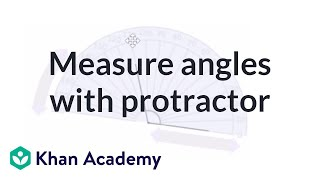By Khan Academy

Let's use our virtual protractor to measure some angles together, shall we?# Measuring angles in degrees | Angles and intersecting lines | Geometry | Khan Academy

##By Khan Academy

Let's come up with an exact way to measure an angle using a protractor. We'll also learn about acute angles. Not to be confused with augly angles :)# Angle basics | Angles and intersecting lines | Geometry | Khan Academy

##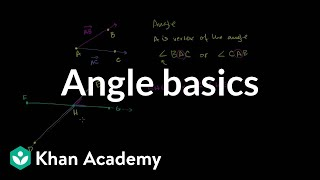By Khan Academy

The naming of an angle is related to the line segments that create it. We'll also learn about the angle's vertex. Come on. Let's do this.# Math Antics - Angle Basics

##By mathantics# Understand Quadrilaterals - Trapezoid, Rhombus, Parallelogram, Rectangle, Square, Kite - 

##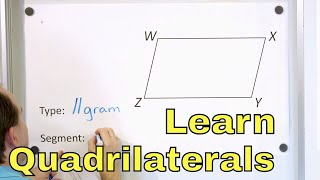By Math and Science

Quality Math And Science Videos that feature step-by-step example problems!# Learn Regular Polygons, Rhombus, Triangle, Parallelogram, Pentagon, Hexagon, Square - [5-9-3]

##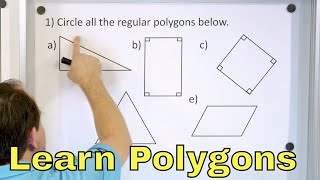By Math and Science

Quality Math And Science Videos that feature step-by-step example problems!# ALL OF GRADE 9 MATH IN 60 MINUTES!!! (exam review part 2)

##By Lumos Learning

Here is a great exam review video reviewing all of the main concepts you would have learned in the MPM1D grade 9 academic math course. The video is divided in to 3 parts. This is part 2: Linear Relations. In this video you will review everything there is to know about y=mx+b, scatterplots, and distance time graphs.